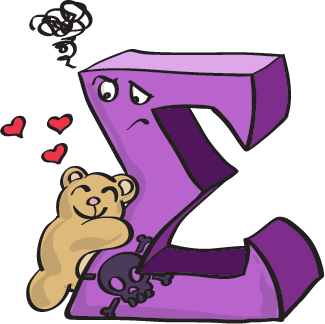Arithmetic Series
4 years ago
kymberlie.hare
Save
Edit
Host a game
Live GameLive
Homework
Solo Practice
Practice6 QuestionsShow answers
• Question 1
180 seconds
Q. Find S14
7, 2, -3, ...
-812
119
-945
-357
• Question 2
180 seconds
Q. The sequence 28, 34, 40, 46, 52, ..., 88 has 11 terms. Evaluate the related series.
550
638
610
288
• Question 3
180 seconds
Q. Find Sn for the given series:
a1=22
d=-8
n=21
1911
-1449
-2436
-1218
• Question 4
180 seconds
Q. Find the sum
24+48+72+...+480
5280
9576
10080
5040
• Question 5
180 seconds
Q. Find the missing terms of the arithmetic sequence:
145, ___, ___, ___, 205
160, 175, 190
165, 185, 195
155, 165, 175
190, 175, 160
• Question 6
180 seconds
Q. Find how many terms are in the series below:
7+13+19+25...=1044